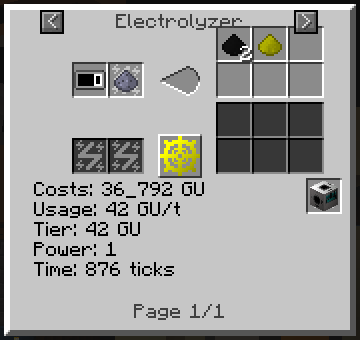# Petroleum Coke Dust weight / electrolyzer recipe

I was browsing through NEI today and I noticed something strange about `Petroleum Coke`.

`Petroleum Coke` has a chemical formula of C2S. Assuming it follows the usual pattern you see in GregTech, that should mean that 3 units of `Petroleum Coke` contain 2 units of `Carbon` and 1 unit of `Sulfur`.

As expected, there’s an electrolyzer recipe that allows you to separate `Petroleum Coke` into 2x `Carbon Dust` and 1x `Sulfur Dust`, however it only takes 1x of `Petroleum Coke Dust`, not 3x as expected. Is this an oversight?Another thing I noticed is that 3 units of `Petroleum Coke` is somehow lighter than the 2 units of Carbon and 1 unit of Sulfur it’s made up of. Here’s my math:

1 unit of `Petroleum Coke` is 103 + 2/9 = 103.222… kg.
3 units of `Petroleum Coke` is 309 + 2/3 = 309.666… kg.

1 unit of `Carbon` is 251 + 8/9 = 251.888… kg.
2 units of `Carbon` is 503 + 7/9 = 503.777… kg.
1 unit of `Sulfur` is 229 + 2/3 = 229.666… kg.

2 units of `Carbon` + 1 unit of `Sulfur` = 733+4/9 = 733.444… kg.

If 3 units of `Petroleum Coke` = 2 units of `Carbon` + 1 unit of `Sulfur`,
then 1 unit of `Petroleum Coke` should equal (733+4/9)kg / 3 = (244 + 13/27)kg = 244.481481… kg, not 103.222… kg like it currently is, shouldn’t it?

2 Likes

Yeah this is a complicated thing, it is intended to be 1 → 3 in the Recipe, I can set the divider manually and do so for a lot of Materials actually.

In case of coal I had to set some weight parameters manually to keep some semblance of consistency in one way, while sacrificing it in the way you described too.

2 Likes